# How to Write a Chemical Compound Formula?

02.12.2022School educationHigher Education

In fact, this is a simple task if you have certain knowledge and understand certain algorithms. But those who are just beginning to learn chemistry may experience various kinds of difficulties, for example, how to display different properties of constituents in a formula.

We will tell you how to do it yourself and cope with the learning problems you face in case something is unclear.

## What is a Compound in Chemistry?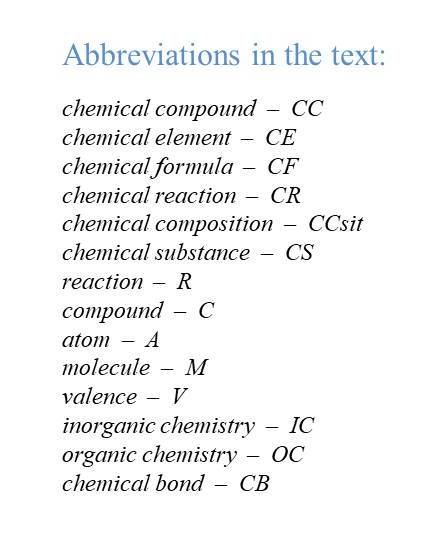A CC is a substance which molecules consist of As of two or more different CE interconnected by one or another type of CB. A C has a certain CCsit and can be attributed a precise CF. Both elements of the same species and elements of different species can combine into CCs. Unlike mixtures of CEs, a CC has qualitatively new properties that are not reduced to the properties of the CEs that are part of it.

CCs are obtained as a result of CRs, during which heat can be released (exothermic R) or absorbed (endothermic R). What does a compound look like? The concept of a CC is idealized: unlike real chemicals, most of which are mixtures of different CCs. Usually, a structural unit of a CC is a molecule, the CCsit and structure of which can be determined by a certain CF. CSs, which have in their composition practically only one CC, are called pure.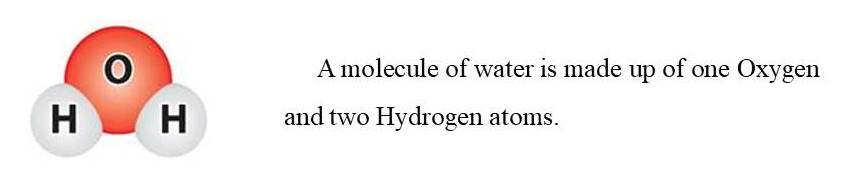However, there are substances of non-molecular structure and even compounds of variable composition (bertolides). In such cases, when a structural unit is difficult to determine or difficult to imagine, a CCsit is expressed by a formula unit.

Since a C includes various CEs, substances are referred to as complex. There are incomparably more complex substances than simple ones. If simple substances are now known to be more than 500 (this is more than the number of elements due to allotropy), then there are more than 50 thousand complex substances in IC, and about 3 million in OC.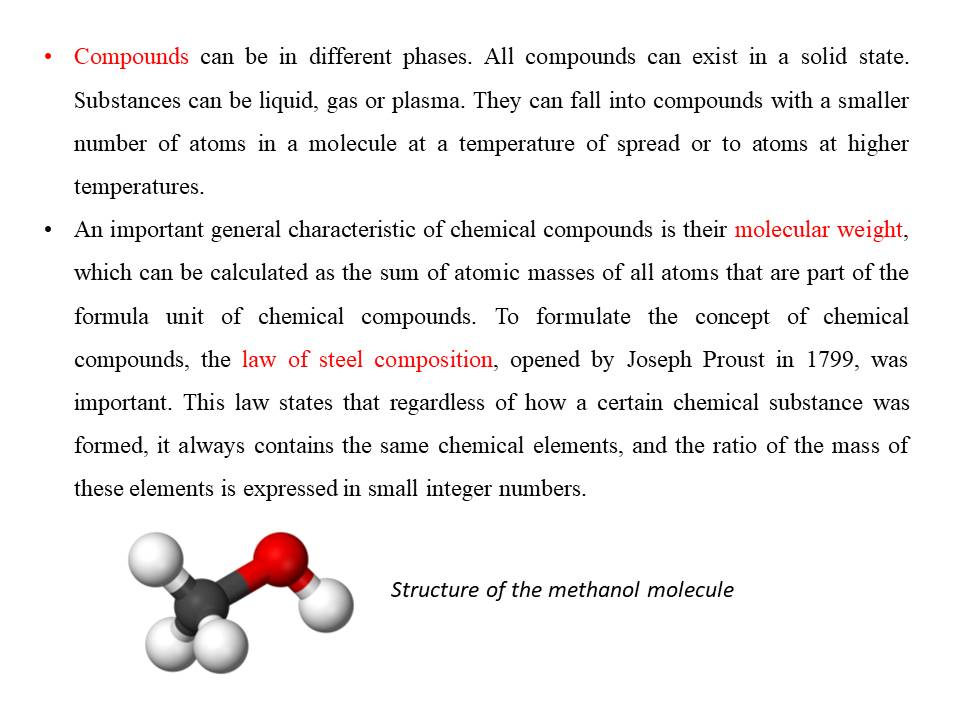This topic is rather multifaceted and includes studying the properties of different compounds. You can get the knowledge on your own, but if you don't have a solid base in chemistry, it will be very difficult for you. Especially with the derivation of formulas. So you can improve your level with additional courses or one-on-one tutoring classes, which you can choose from the UpskillsTutor site.

## How to Write Chemical Formulas?

A conditional entry of a composition of a substance using symbols of CEs and indexes is called a formula. It displays the composition of Cs. Let us define two key terms.

• Index is the number of As that are connected to each other by a CB.
• Coefficient shows the number of individual particles (atoms, molecules, ions). For example, two Hydrogen molecules are 2Н2, two Hydrogen atoms are 2H.

Let us take an example of an apatite molecule Ca3(PO4)2. In it, the numbers 3, 4, and 2 are indexes that show how many As are connected to each other as a whole. If you put 5 ahead, then this is the coefficient.

Consider the algorithm of writing formula for different substances.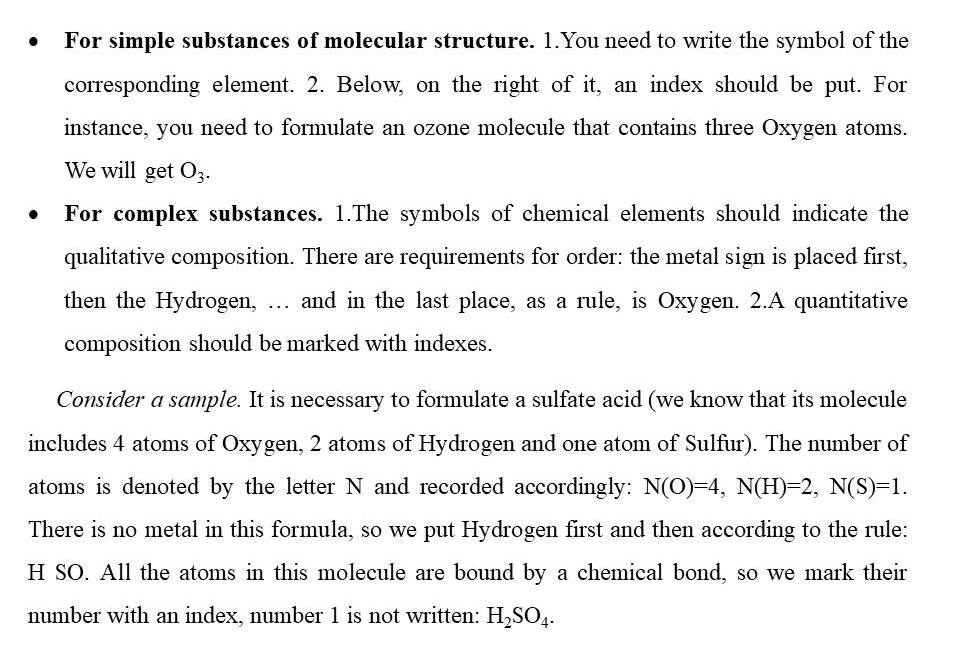• For substances of non-molecular structure. A CF coincides with the symbol for writing a CE. For example, the CF of silicon is Si (it is the symbol of the CE Silicium).

Brackets are also used when assembling formulas. They distinguish groups of As that are repeated. The side index near the brackets relates to each CE recorded in brackets.

## Searching а Compound Formula by Valence

If valences of two elements are known, then it is possible to formulate a formula of their Cs.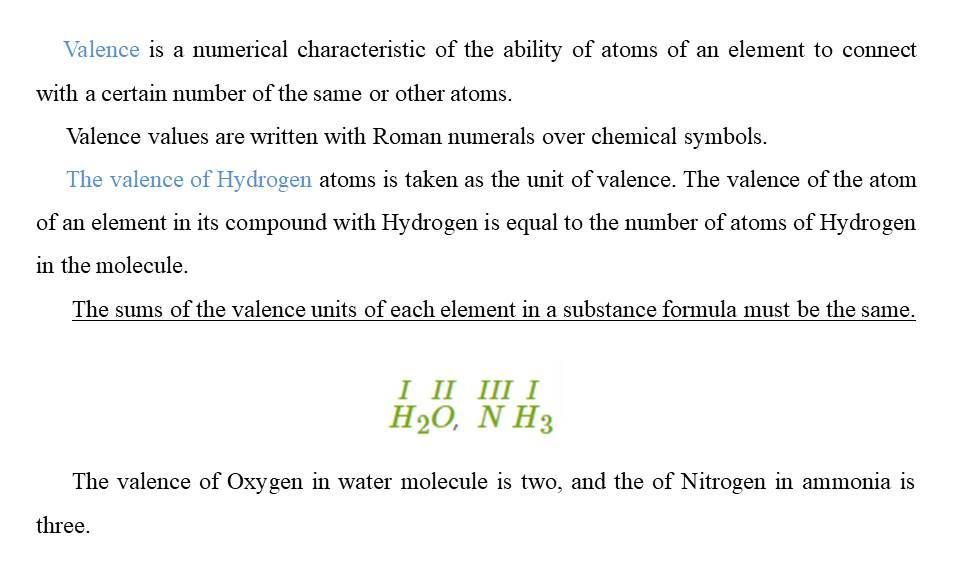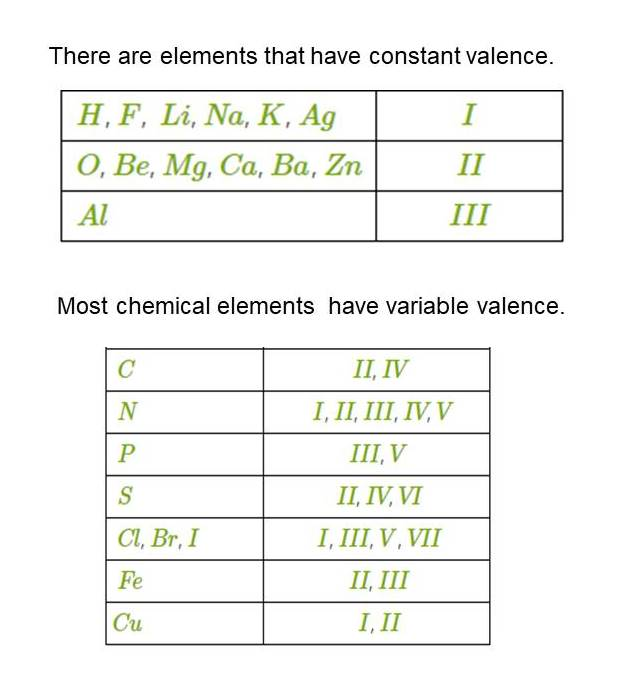Consider a sample problem showing how to write CFs if a valence of one of the elements is known.

It is necessary to write a formula for the chlorine C, the valence of which is equal to VII with Oxygen.

1. Write the symbols of CEs next to each other. Specify the valences above them. The valence of Oxygen is constant and is equal to II.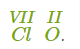2. Find the smallest common multiple of valences, we write it over the formula: 7×2=14.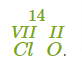3. Divide the smallest common multiple by the valence of each element: 14:7=2, 14:2=7.
4. We receive such indexes in the formula of C: Cl2O7.

If you consider the introduction to this material (compound in chemistry in general), the explanations and examples may seem understandable to you. However, in order to study the topic in depth and solve more complex problems, the advice of a teacher will be required.

Classes with a tutor are an opportunity to spend productive training time, quickly eliminate gaps in knowledge and get answers to all your questions. For children, this is also an opportunity to recharge with additional motivation.

Just think of the benefits that give you classes with a tutor:

• a personal curriculum;
• your training goal is completely considered;
• many practical tasks, a lot of experimentation;
• convenient study conditions and timetable.

### Read more: What is the GRE Analytical Writing Assessment?

Rate the article

Rate: 5 of 5

Based on reviews from 2 users

Need a tutor?

Choose the best teachers on the UpskillsTutor service!

### How to Write a Chemical Compound Formula?

#### What is a Compound in Chemistry?

A CC is a substance which molecules consist of As of two or more different CE interconnected by one or another type of CB… Read more on UpskillsTutor

#### How to Write Chemical Formulas?

A conditional entry of a composition of a substance using symbols of CEs and indexes is called a formula. It displays the composition of Cs… Read more on UpskillsTutor
;